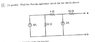# Basic EE Norton Equivalent circuit

• Engineering
ihavaquestion

## Homework Statement

Find norton equivalent circuit

Rn=Rth

## The Attempt at a Solution

Ok i got my R(n)=34 ohms because after you turn off independent sources the resistors are in series, then I short circuited a to be and I'm trying to use mesh analysis to solve for I(n)
the problem arises with the 2 amp current source does that make I2 and I3 2 amps? help!?

#### Attachments

•3.jpg
16.5 KB · Views: 299
Last edited:

CEL

## Homework Statement

Find norton equivalent circuit

Rn=Rth

## The Attempt at a Solution

Ok i got my R(n)=34 ohms because after you turn off independent sources the resistors are in series, then I short circuited a to be and I'm trying to use mesh analysis to solve for I(n)
the problem arises with the 2 amp current source does that make I2 and I3 2 amps? help!?

You can write the mesh equations for the circuit and calculate the current in ab.
Or you can use superposition: cancel one of the current sources and calculate the current, then activate that source and cancel the other. The total sum of the two partial currents. current is the

Mentor
the problem arises with the 2 amp current source does that make I2 and I3 2 amps? help!?

You didn't label the diagram with mesh currents, so I'll guess that you have three meshes numbered from left to right in the circuit. For clockwise mesh currents i1, i2, i3, the 2A independent source will make i3 - i2 = 2A.

Note that you could also solve the problem pretty easily using superposition: you get a couple of straightforward current dividers to deal with.

ihavaquestion